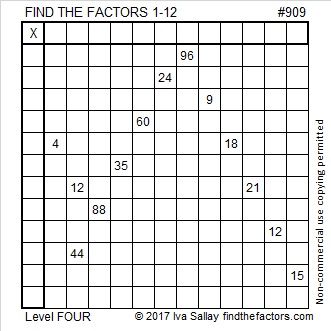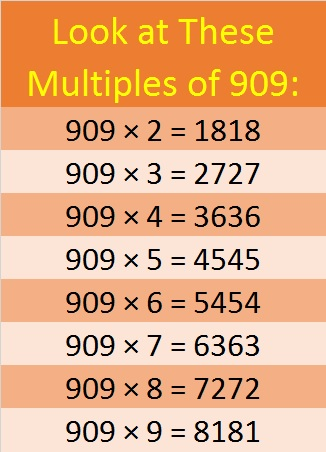# 909 and Level 4

909 is a palindrome in base 10 because 9(100) + 0(10) + 9(1) = 909.

It is also palindrome 757 in BASE 11 because 7(121) + 5(11) + 7(1) = 909.Print the puzzles or type the solution on this excel file: 12 factors 905-913

Here’s a little more about the number 909:

30² + 3² = 909

909 is the hypotenuse of Pythagorean triple 180-891-909, which is 9 times (20-99-101) and can be calculated from 2(30)(3), 30² – 3², 30² + 3²

As you know, 909 is made with 9’s and 0’s. OEIS.org informs us that 909 times 2, 3, 4, 5, 6, 7, 8, or 9 do NOT contain even one 9 or 0. That’s a little spooky, but see for yourself:• 909 is a composite number.
• Prime factorization: 909 = 3 × 3 × 101, which can be written 909 = 3² × 101
• The exponents in the prime factorization are 2 and 1. Adding one to each and multiplying we get (2 + 1)(1 + 1) = 3 × 2  = 6. Therefore 909 has exactly 6 factors.
• Factors of 909: 1, 3, 9, 101, 303, 909
• Factor pairs: 909 = 1 × 909, 3 × 303, or 9 × 101
• Taking the factor pair with the largest square number factor, we get √909 = (√9)(√101) = 3√101 ≈ 30.14962686